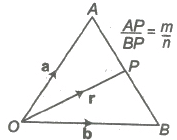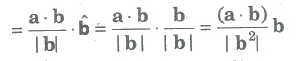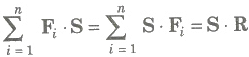JEE  >  Important Formulas: Section Formula & Scalar Product of Two Vectors

# Important Formulas: Section Formula & Scalar Product of Two Vectors - Mathematics (Maths) Class 12 - JEE

Section Formula

Let A and B be two points with position vectors a and b, respectively and OP= r.

(i) Let P be a point dividing AB internally in the ratio m : n. Then,

r = m b + n a / m + nAlso, (m + n) OP = m OB + n OA

(ii) The position vector of the mid-point of a and b is a + b / 2.

(iii) Let P be a point dividing AB externally in the ratio m : n. Then,

r = m b + n a / m + n

Position Vector of Different Centre of a Triangle

(i) If a, b, c be PV’s of the vertices A, B, C of a ΔABC respectively, then the PV of the centroid G of the triangle is a + b + c / 3.

(ii) The PV of incentre of ΔABC is (BC)a + (CA)b + (AB)c / BC + CA + AB

(iii) The PV of orthocentre of ΔABC is

a(tan A) + b(tan B) + c(tan C) / tan A + tan B + tan C

Scalar Product of Two Vectors

If a and b are two non-zero vectors, then the scalar or dot product of a and b is denoted by a * b and is defined as a * b = |a| |b| cos θ, where θ is the angle between the two vectors and 0 < θ < π .

(i) The angle between two vectors a and b is defined as the smaller angle θ between them, when they are drawn with the same initial point.

Usually, we take 0 < θ < π.Angle between two like vectors is O and angle between two unlike vectors is π .

(ii) If either a or b is the null vector, then scalar product of the vector is zero.

(iii) If a and b are two unit vectors, then a * b = cos θ.

(iv) The scalar product is commutative

i.e., a * b= b * a

(v) If i , j and k are mutually perpendicular unit vectors i , j and k, then

i * i = j * j = k * k =1

and i * j = j * k = k * i = 0

(vi) The scalar product of vectors is distributive over vector addition.

(a) a * (b + c) = a * b + a * c (left distributive)
(b) (b + c) * a = b * a + c * a (right distributive)

Note Length of a vector as a scalar product

If a be any vector, then the scalar product

a * a = |a| |a| cosθ ⇒ |a|2 = a2 ⇒ a = |a|

Condition of perpendicularity a * b = 0 <=> a ⊥ b, a and b being non-zero vectors.

Important Points to be Remembered

(i) (a + b) * (a – b) = |a|22 – |b|2

(ii) |a + b|2 = |a|22 + |b|2 + 2 (a * b)

(iii) |a – b|2 = |a|22 + |b|2 – 2 (a * b)

(iv) |a + b|2 + |a – b|2 = (|a|22 + |b|2) and |a + b|2 – |a – b|2 = 4 (a * b)

or a * b = 1 / 4 [ |a + b|2 – |a – b|2 ]

(v) If |a + b| = |a| + |b|, then a is parallel to b.

(vi) If |a + b| = |a| – |b|, then a is parallel to b.

(vii) (a * b)2 ≤ |a|22 |b|2

(viii) If a = a1i + a2j + a3k, then |a|2 = a * a = a12 + a22 + a32

Or

|a| = √a12 + a22 + a32

(ix) Angle between Two Vectors If θ is angle between two non-zero vectors, a, b, then we have

a * b = |a| |b| cos θ

cos θ = a * b / |a| |b|

If a = a1i + a2j + a3k and b = b1i + b2j + b3k

Then, the angle θ between a and b is given by

cos θ = a * b / |a| |b| = a1b1 + a2b2 + a3b3 / √a12 + a22 + a32 √b12 + b22 + b32

(x) Projection and Component of a Vector

Projection of a on b = a * b / |a|

Projection of b on a = a * b / |a|

Vector component of a vector a on bSimilarly, the vector component of b on a = ((a * b) / |a2|) * a

(xi) Work done by a Force

The work done by a force is a scalar quantity equal to the product of the magnitude of the force and the resolved part of the displacement.

∴ F * S = dot products of force and displacement.

Suppose F1, F1,…, Fn are n forces acted on a particle, then during the displacement S of the particle, the separate forces do quantities of work F1 * S, F2 * S, Fn * S.

Total workdone isHere, system of forces were replaced by its resultant R.

The document Important Formulas: Section Formula & Scalar Product of Two Vectors | Mathematics (Maths) Class 12 - JEE is a part of the JEE Course Mathematics (Maths) Class 12.
All you need of JEE at this link: JEE

## Mathematics (Maths) Class 12

206 videos|264 docs|139 tests

## Mathematics (Maths) Class 12

206 videos|264 docs|139 testsExplore Courses for JEE examSignup to see your scores go up within 7 days! Learn & Practice with 1000+ FREE Notes, Videos & Tests.
10M+ students study on EduRev
Track your progress, build streaks, highlight & save important lessons and more!
Related Searches

,

,

,

,

,

,

,

,

,

,

,

,

,

,

,

,

,

,

,

,

,

;